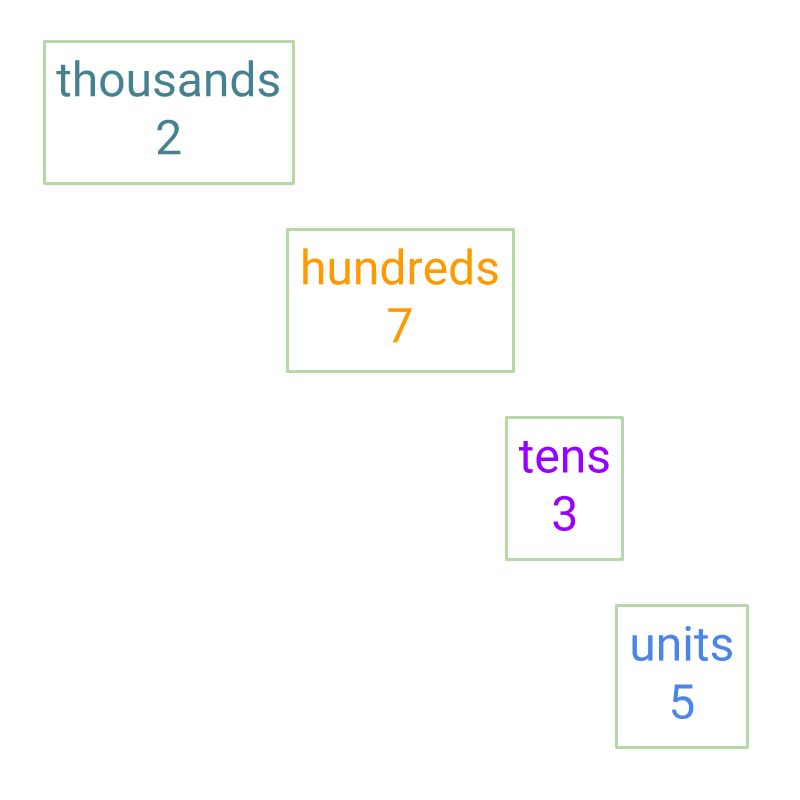maths > wholenumbers

Expanded form of Numbers

what you'll learn...

overview

This page introduces the concept of "number of digits" in a number and 2-digit numbers, 3-digit numbers, etc.

In the previous pages, the numbers were introduced with grouping of

•  10$10$ units to 1$1$ ten,

•  10$10$ tens to 1$1$ hundreds

•  and so on.

That lead to numbers in standard numerical format.

In this page, the understanding of standard numerical format in reverse is explained with the two expanded forms

•  7253=7000+200+50+3$7253 = 7000 + 200 + 50 + 3$

•  = 7$7$ thousands, $2$ hundreds, $5$ tens, 3 units.

Then the two popular systems of large numbers is introduced.

•  Indian system of large numbers.

•  International system of large numbers.

Expanded form of a number

The number $65$ has $2$ digits.

The number $24$ is made of two digits $2$ and $4$. This is another example of a $2$ digit number.

The number $423$, has $3$ digits.

The digits in the number $264$ are $2$, $6$, and $4$. This is another example of a $3$ digit number. This represents

$2$ hundreds

$6$ tens

$4$ units.The expanded form of the given number $2735$.

$2735$

$\quad = 2000 + 700 + 30 + 5$

$\quad = 2$ thousands $+ 7$ hundreds $+ 3$ tens $+ 5$ units

to expand means: to make something large by giving more details. Expanded form of a number provides the place value of each digits of the number.

What is the number $652734$ in expanded form?
The answer is "$600000 + 50000$$+ 2000$$+ 700$$+ 30 + 4$".

Expanded form: provides the place value of each digits of the number.
Eg: $1234 = 1000 + 200 + 30 + 4$

What is the expanded form of $872$?
The answer is '$8$ hundred, $+ 7$ ten, $+ 2$'

What is the expanded form of $46427$?
The answer is $40000 + 6000 + 400 + 20 + 7$
= $4$ ten thousand $+ 6$ thousand $+ 4$ hundred $+ 2$ ten $+ 7$

Indian System

Large Numbers - Indian System :

$1$ - unit

$10$ - ten

$100$ - hundred

$1 , 000$ - thousand

$10 , 000$ - ten thousand

$1 , 00 , 000$ - lakh

$10 , 00 , 000$ - ten lakh

$1 , 00 , 00 , 000$ - crore

International System

Large Numbers - International System :

$1$ - unit

$10$ - ten

$100$ - hundred

$1 , 000$ - thousand

$10 , 000$ - ten thousand

$100 , 000$ - hundred thousand

$1 , 000 , 000$ - million

$10 , 000 , 000$ - ten million

$100 , 000 , 000$ - hundred million

$1 , 000 , 000 , 000$ - billion

Summary

• 2-digit number: A number with only tens and units places.

• 3-digit number: A number with only hundreds, tens, and units places.

Expanded forms of a number

$7253$

$= 7000 + 200 + 50 + 3$

$= 7$ thousands, $2$ hundreds, $5$ tens, 3 units.

$=$ seven thousand, two hundred, fifty three.

•  Indian system of large numbers.
»  $1$ - unit
»  $10$ - ten
»  $100$ - hundred
»  $1 , 000$ - thousand
»  $10 , 000$ - ten thousand
»  $1 , 00 , 000$ - lakh
»  $10 , 00 , 000$ - ten lakh
»  $1 , 00 , 00 , 000$ - crore

•  International system of large numbers.
»  $1$ - unit
»  $10$ - ten
»  $100$ - hundred
»  $1 , 000$ - thousand
»  $10 , 000$ - ten thousand
»  $100 , 000$ - hundred thousand
»  $1 , 000 , 000$ - million

»  $10 , 000 , 000$ - ten million
»  $100 , 000 , 000$ - hundred million
»  $1000 , 000 , 000$ - billion

Outline